# Sorting Numbers Based on their Frequency within a List

In this article, we will learn how to sort the numbers based on their frequency in the range.

We use Frequency to know how often a value is repeating in a range.

To understand the way to sort number based on their frequency within a list, we will take 2 Examples.

Example 1

Let’s take an example and understand how we can sort the data on the basis of frequency.

We have an agent data with number of sales. We want to sort the data as per their frequency of sales number.First we will return the frequency of the numbers, follow below steps:-

• In cell C2 EnterCOUNTIF function.
• =COUNTIF(\$B\$2:\$B\$24,B2), press enter
• And copy the same formula in the range C2:C24.To sort the data as per the frequency.

• Select the data A1:C24.
• Go to Data tab > Click on Sort option.
• Sort dialog box will appear.
• From the Sort by option> Select Frequency.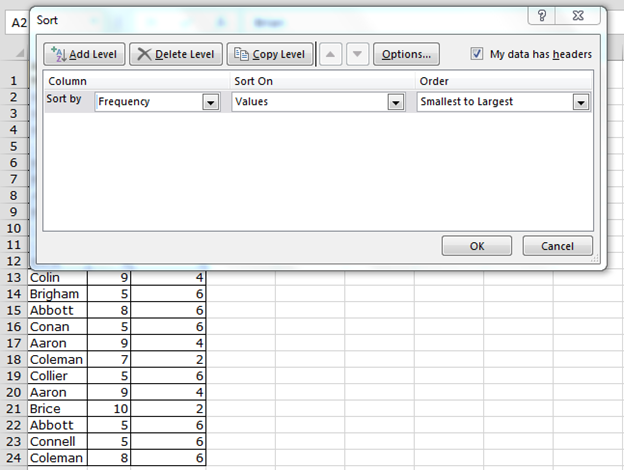• In Sort on option > select values.
• In order > Largest to smallest.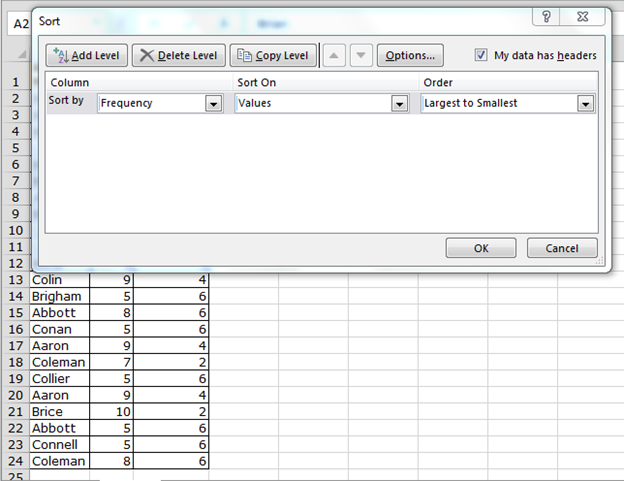• Click on ok.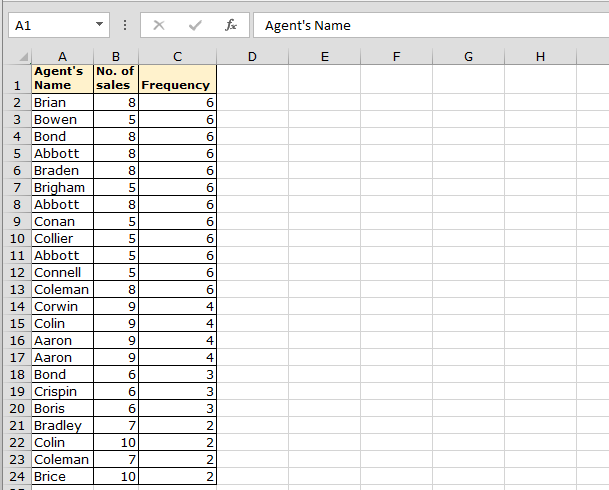Example 2

Now we have product list, in which we want to see that which product is selling more through agents.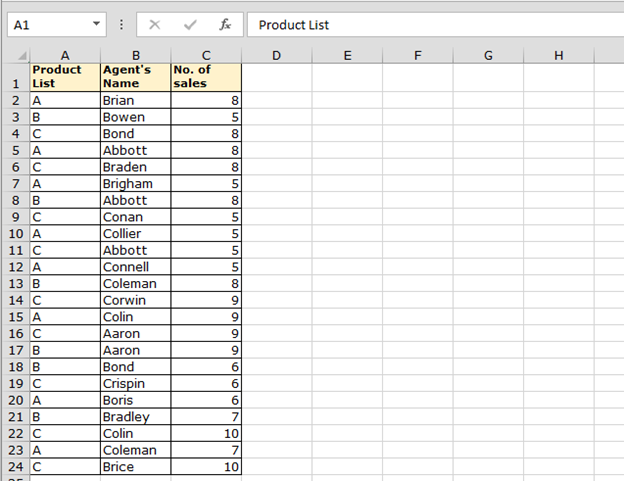First we will return the frequency of product:-

• Enter the formula in Cell D2.
• =COUNTIF(\$A\$2:\$A\$24,A2)
• Copy the formula in the range D3:D24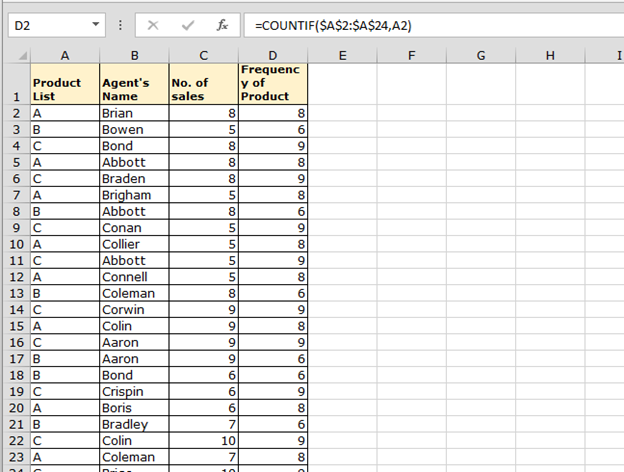We will sort the data according to the frequency of the product. Follow below steps:-

• Select the data range.
• Go to Data tab > Sort option.
• Sort dialog box will appear.
• In the sort by, select Frequency of product.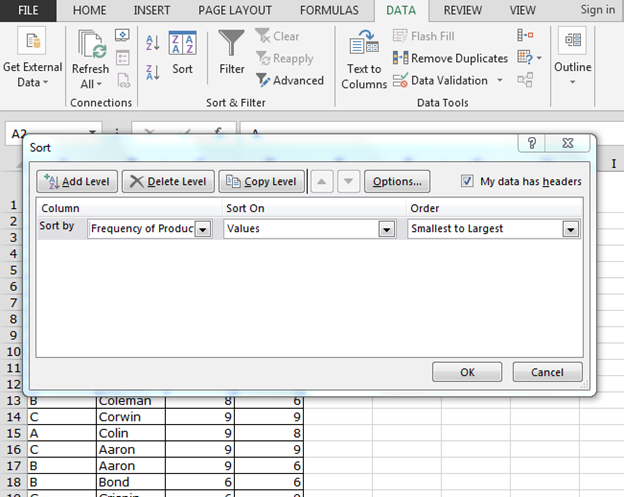• In the sort on option > select values.
• In the order option > select largest to smallest.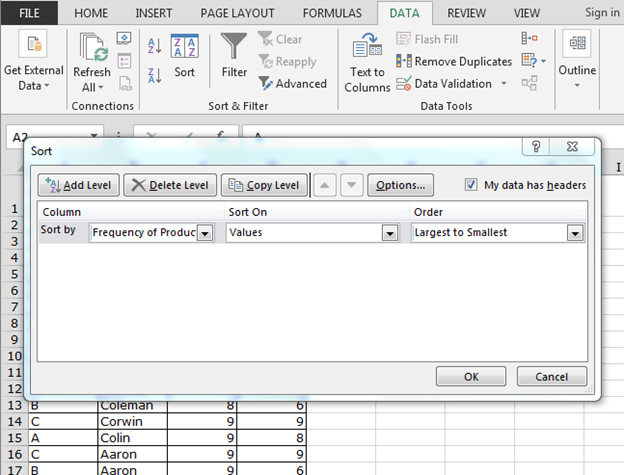• Click on ok.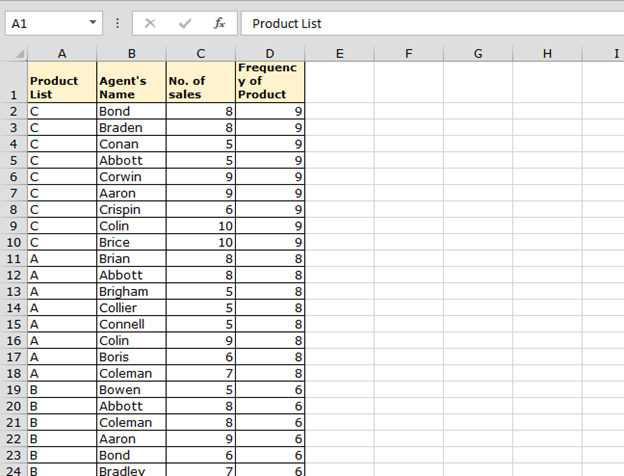Conclusion: -In Excel, we can sort data in any of the ways provided that our requirement is clear.

Taken away points:-

• Sort data according to the frequency of number.
• Sort data according to the frequency of text.